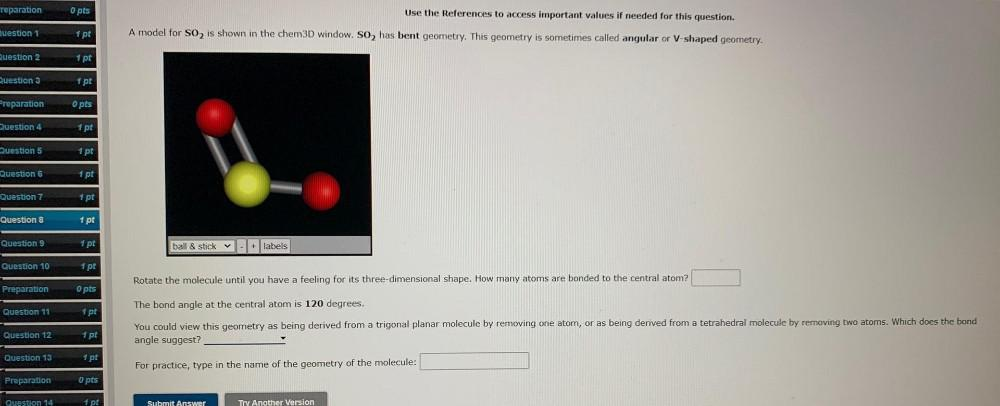Question:

# reparation O pts Use the References to access important values if needed for this question. Ruestion 1 1 pt A model for S02 is sreparation O pts Use the References to access important values if needed for this question. Ruestion 1 1 pt A model for S02 is shown in the chem3D window. So, has bent geometry. This geometry is sometimes called angular or V-shaped geometry. Question 2 1 pt Question 3 1 pt Preparation O pts Question 4 1 pt Question 5 1 pt Question 6 1 pt Question 7 1 pt Question 8 1 pt Question 9 1 pt ball & stick + labels Question 10 1 pt Rotate the molecule until you have a feeling for its three-dimensional shape. How many atoms are bonded to the central atom? Preparation O pts Question 11 1 pt The bond angle at the central atom is 120 degrees. You could view this geometry as being derived from a trigonal planar molecule by removing one atom, or as being derived from a tetrahedral molecule by removing two atoms. Which does the bond angle suggest? Question 12 1 pt Question 13 1 pt For practice, type in the name of the geometry of the molecule: Preparation opts Question 14 1 pt Submit Answer Try Another Version